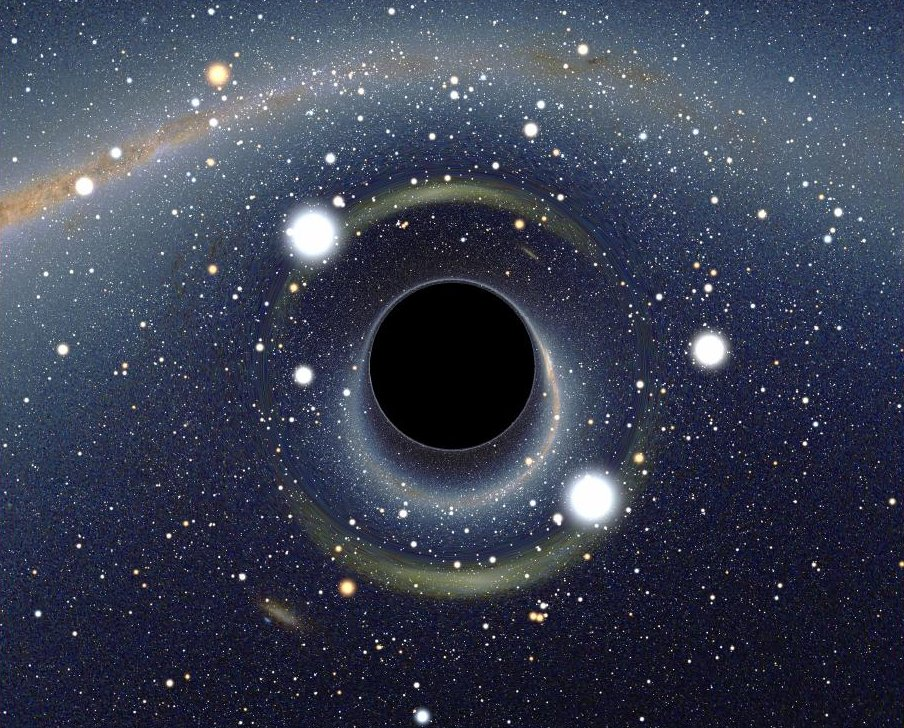# Differential Geometry III — Lorentzian Geometry

Prof. Bernd Ammann, Office no. 119This picture arose from computer calculations using basic properties of Lorentzian manifolds. It represents a black hole.
The image was obtained from the web page linked here
Picture created by Alain Riazuelo, IAP/UPMC/CNRS under the license CC-BY-SA 3.0.

# Content

In this lecture we want to deepen our knowledge about semi-Riemannian and in particular Lorentzian manifolds. The precise content will be fixed a bit later, but it is likely to be a choice out of the following:
• Where do the Einstein equations come from? The Einstein equations as stationary points of a variational problem
• gravitational waves
• special solutions of Einstein's equations, e.g. the Kerr solution for rotating black holes
• sagemath/python tools to do calculations in general relativity
• the positive mass theorem
• wave equations on Lorentzian manifolds, leading to quatization of fields
• solving the Einstein equations as a pde
• more about causality, Cauchy hypersurfaces, global hyperbolicity
• The Yamabe problem

## Prerequisites

Analysis I-IV, Lineare Algebra I+II, differential geometry I. Helpful is the lecture differential geometry II, but as the topics are pretty disjoint, the gaps could be compensated by reading some literature.

## Time and Location

Monday and Wednesday 8.15-10.00
Monday 8.15-10.00 in M102 and Wednesday 8-15-10.00 in M101
Please register on GRIPS to get the latest news and information about the exercises.

## Lecture Notes

Partial lecture Notes are available here. Please assume that they will not be continued. In parts of the lecture I use excerpts from the the Ammann-Bär lecture about the Yamabe problem (German language)

### Exercise Sheets

(some links are not active yet)
All Exercise sheets in one file

## Literature

### Literature about the physics associated to the subject

#### Books

• M. do Carmo, Riemannian Geometry, Birkhäuser
• Cheeger, Ebin, Comparison theorems in Riemannian Geometry
• F. Warner, Foundations of differentiable manifolds and Lie groups, Springer
• T. Sakai, Riemannian Geometry, Transl. Math. Monogr., AMS
• W. Kühnel, Differentialgeometrie, Vieweg
• J. Lee, Introduction to topological manifolds, Springer
• J. Lee, Introduction to smooth manifolds, Springer
• J. Lee, Riemannian manifolds, Springer